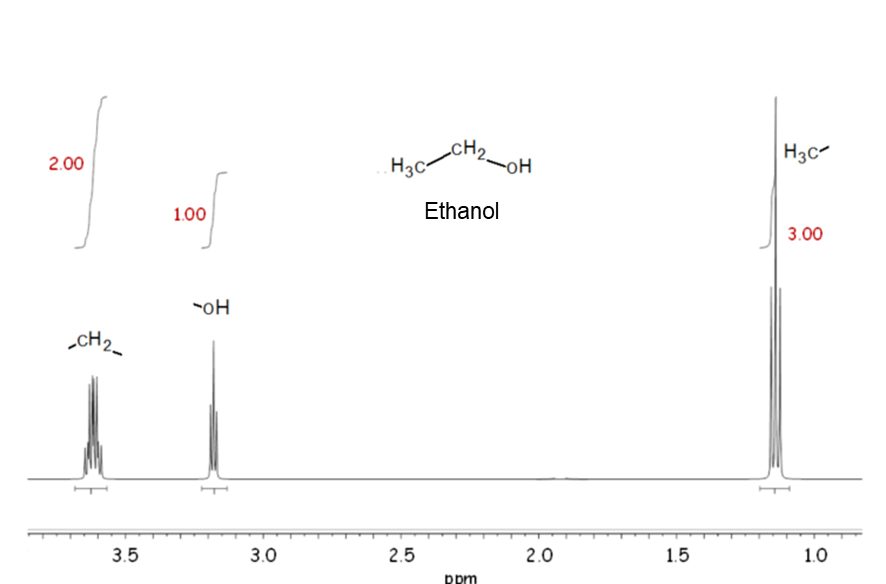# Nuclear Magnetic Resonance (NMR)

As the NMR spectrum can estimate the molecular structure based on the information such as chemical shift values of signals, coupling, and intramolecular integration ratio, it is used for qualitative analysis, mainly for molecular structure analysis in the fields of organic chemistry and natural products chemistry.
When electromagnetic waves are irradiated on a substance placed in the magnetic field, electromagnetic waves (radio waves) of the frequency specific to the atomic nucleus in the substance are absorbed and released; this is called nuclear magnetic resonance (NMR). NMR spectroscopy (simply called NMR) is a method to record the frequency of electromagnetic waves absorbed per the characteristics of the atomic nucleus as a function of the absorption peak intensity, and its record is NMR spectrum. In real compounds, even if the type of nuclei (1H and 13C) is the same, the frequency at which resonant absorption occurs differs if the surrounding chemical environment is different. This is attributed to the increase or decrease in the strength of the magnetic field caused by shielding the main magnetic field by a small magnetic field of the electrons around the nucleus or adjacent nucleus. Such a difference in resonance frequency is called chemical shift; this difference in frequency is marginal, but information on compounds can be obtained by precisely measuring this marginal difference.
There are two types of NMR: qualitative NMR for molecular structure analysis and quantitative NMR (qNMR) for determining the compound purity by utilizing the characteristics of NMR that the ratio of the number of atomic nuclei in the compound corresponds to the peak area ratio.
We offer solvents, equipment, and reference materials that can be used for qualitative and quantitative NMR analysis.

### Information obtained from NMR spectra#### Chemical Shift

The horizontal axis of the NMR spectrum shows the resonance frequency, which changes based on the chemical environment of each nucleus. This is called a chemical shift, which is usually expressed in frequency units (Hz). The resonance frequency is directly proportional to the field strength of a spectrometer used, and this leads to different resonance frequency for different field strength. This field dependence can be removed when the chemical shifts are expressed in terms of the dimensional unit delta, δ, defined by following equation.As a result, chemical shift is expressed in the unit of parts per million (ppm).

#### Coupling-pattern and spin-spin coupling constant

When nuclei with different chemical environments exist in the vicinity, they interact with each other via the chemical bond axis, resulting in energy-level splitting. This phenomenon is called a spin-spin coupling. The splitting interval is described as a spin-spin coupling constant or J value. Since the coupling nuclei share the same J value, it shows an index to know which nuclei are close to each other.

#### Signal area

In NMR, the signal area (more precisely, the ratio of signal area) is proportional to the number of nuclei. Therefore, in the 1H NMR spectrum of ethanol shown in the example, there are three resonances due to three different types of hydrogen, and the ratio of signal area is 3: 2: 1.

877-714-1920